Python处理的数据类型有哪些？白羽 2018-07-03 来源 ：网络 阅读 142 评论 0

```Python 是一门运用很广泛的语言，自动化脚本、爬虫，甚至在深度学习领域也都有 Python 的身影。作为一名前端开发者，也了解 ES6 中的很多特性借鉴自 Python (比如默认参数、解构赋值、Decorator等)，同时本文会对 Python 的一些用法与 JS 进行类比。不管是提升自己的知识广度，还是更好地迎接 AI 时代，Python 都是一门值得学习的语言。

· 数字[整数(int)、浮点型(float)、长整型(long)、复数(complex)]
· 字符串(str)
· 布尔值(bool)
· 空值(None)

Python

int('3') # 3
str(3.14) # '3.14'
float('3.14') # 3.14
# 区别于 JS 只有 Number 一种类型，Python 中数字中的不同类型也能相互强制转换
float(3) # 3.0
bool(3) # True
bool(0) # False

Python

1 + 1.0 # 2.0
1 + False # 1
1.0 + True # 2.0
# 区别于 JS 的 String + Number = String, py 中 str + int 会报错
1 + '1' # TypeError: cannot concatenate 'str' and 'int' objects

Python

type(1.3) == float # True
isinstance('a', str) # True
isinstance(1.3, int) # False
isinstance(True, bool) # True
isinstance([], list) # True
isinstance({}, dict) # True

list 类型
Python 中 List 类型类似于 JS 中的 Array,
Python

L = [1, 2, 3]
print L[-1] # '3'

L.append(4) # 末尾添加元素
print L # [1, 2, 3, 4]

L.insert(0, 'hi') # 指定索引位置添加元素
print L # ['hi', 1, 2, 3, 4]

L.pop() # 末尾移除元素 L.pop(2) ?????? 2 ???
print L # ['hi', 1, 2, 3]

tuple 类型
tuple 类型是另一种有序的列表，中文翻译为“ 元组 ”。tuple 和 list 非常类似，但是，tuple 一旦创建完毕，就不能修改了。
Python

t = (1, 2, 3)
print t # 1
t = 11 # TypeError: 'tuple' object does not support item assignment

t = (1)
print t # 1  t 的结果是整数 1

t = (1,) # 为了避免出现如上有歧义的单元素 tuple，所以 Python 规定，单元素 tuple 要多加一个逗号“,”
print t # (1,)

dict 类型
Python 中的 dict 类型类似于 JS 中的 {} (最大的不同是它是没有顺序的), 它有如下特点:
· 查找速度快 (无论 dict 有 10 个元素还是 10 万个元素，查找速度都一样)
· 占用内存大 (与 list 类型相反)
· dict 中的 key 不能重复
· dict 中存储的 key-value 序对是没有顺序的
Python

d = {
'a': 1,
'b': 2,
'c': 3
}

print d # {'a': 1, 'c': 3, 'b': 2}  可以看出打印出的序对没有按正常的顺序打出

# 遍历 dict
for key,value in d.items():
print('%s: %s' % (key,value))
# a: 1
# c: 3
# b: 2

set 类型

· set 存储的元素和 dict 的 key 类似，必须是不变对象
· set 存储的元素也是没有顺序的
Python

s = set(['A', 'B', 'C', 'C'])
print s # set(['A', 'C', 'B'])

print s # set(['A', 'C', 'B', 'D'])

s.remove('D')
print s # set(['A', 'C', 'B'])

Python 中的迭代

Python

L = ['apple', 'banana', 'orange']
for index, name in enumerate(L):  # enumerate() 函数把 ['apple', 'banana', 'orange'] 变成了类似 [(0, 'apple), (1, 'banana'), (2, 'orange')] 的形式
print index, '-', name

# 0 - apple
# 1 - banana
# 2 - orange

Python

d = { 'apple': 6, 'banana': 8, 'orange': 5 }
print d.values() # [6, 8, 5]
for v in d.values()
print v
# 6
# 8
# 5

Python

d = { 'apple': 6, 'banana': 8, 'orange': 5 }
for key, value in d.items()
print key, ':', value
# apple : 6
# banana: 8
# orange: 5

Python 提供的切片操作符类似于 JS 提供的原生函数 slice()。有了切片操作符，大大简化了一些原来得用循环的操作。
Python

L = ['apple', 'banana', 'orange', 'pear']
L[0:2] # ['apple', 'banana'] 取前 2 个元素
L[:2] # ['apple', 'banana'] 如果第一个索引是 0，可以省略
L[:] # ['apple', 'banana', 'orange', 'pear'] 只用一个 : ，表示从头到尾
L[::2] # ['apple', 'orange'] 第三个参数表示每 N 个取一个，这里表示从头开始，每 2 个元素取出一个来

Python

L = []
for x in range(1, 11):
L.append(x * x)

Python

# 把要生成的元素 x * x 放到前面，后面跟 for 循环，就可以把 list 创建出来
[x * x for x in range(1, 11)]
# [1, 4, 9, 16, 25, 36, 49, 64, 81, 100]

Python

[x * x for x in range(1, 11) if x % 2 == 0]
# [4, 16, 36, 64, 100]

for 循环可以嵌套，因此，在列表生成式中，也可以用多层 for 循环来生成列表。
Python

[m + n for m in 'ABC' for n in '123']
# ['A1', 'A2', 'A3', 'B1', 'B2', 'B3', 'C1', 'C2', 'C3']

Python 函数

JS 中 ES6 的 默认参数正是借鉴于 Python，用法如下：
Python

def greet(name='World'):
print 'Hello, ' + name + '.'

greet() # Hello, World.
greet('Python') # Hello, Python.

Python

def fn(*args):
print args

fn()  # ()
fn('a') # ('a',)
fn('a', 'b') # ('a', 'b')

Python 解释器会把传入的一组参数组装成一个 tuple 传递给可变参数，因此，在函数内部，直接把变量 args 看成一个 tuple 就好了。

Python 中常用的函数 (map、reduce、filter) 的作用和 JS 中一致，只是用法稍微不同。
· map 函数: 接收一个函数 f 和一个 list，并通过把函数 f 依次作用在 list 的每个元素上，得到一个新的 list 并返回。
Python

def f(x):
return x * x
print map(f, [1, 2, 3, 4, 5, 6, 7, 8, 9]) # [1, 4, 9, 16, 25, 36, 49, 64, 81]

· reduce 函数: 接收一个函数 f 和一个 list(可以接受第三个值作为初始值)，reduce() 对 list 的每个元素反复调用函数 f，并返回最终结果值。
Python

def f(x, y):
return x * y

reduce(f, [1, 3, 5]) # 15

· filter 函数: 接收一个函数 f 和一个list，这个函数 f 的作用是对每个元素进行判断，返回 True或 False，filter() 根据判断结果自动过滤掉不符合条件的元素，返回由符合条件元素组成的新 list。
Python

def is_odd(x):
return x % 2 == 1

filter(is_odd, [1, 4, 6, 7, 9, 12, 17]) # [1, 7, 9, 17]

Python

map(lambda x: x * x, [1, 2, 3, 4, 5, 6, 7, 8, 9]) # [1, 4, 9, 16, 25, 36, 49, 64, 81]

Python

def f(x):
return x * x

Python

# 希望一次返回3个函数，分别计算1x1,2x2,3x3:
def count():
fs = []
for i in range(1, 4):
def f():
return i * i
fs.append(f)
return fs

f1, f2, f3 = count() # 这种写法相当于 ES6 中的解构赋值
print f1(), f2(), f3() # 9 9 9

Python

def count():
fs = []
for i in range(1, 4):
def f(j):
def g(): # 方法一
return j * j
return g
r = f(i)
fs.append(r)
return fs

f1, f2, f3 = count()
print f1(), f2(), f3() # 1 4 9

Python

def count():
fs = []
for i in range(1, 4):
def f(j = i): # 方法二
return j * j
fs.append(f)
return fs

f1, f2, f3 = count()
print f1(), f2(), f3() # 1 4 9

decorator 装饰器
ES6 的语法中的 decorator 正是借鉴了 Python 的 decorator。decorator 本质上就是一个高阶函数，它接收一个函数作为参数，然后返回一个新函数。

Python

@Post('/rider/detail')  // URL 路由
@log()                   // 打印日志
@ResponseBody
public async getRiderBasicInfo(
@RequestBody('riderId') riderId: number,
@RequestBody('cityId') cityId: number,
) {
const result = await this.riderManager.findDetail(cityId, riderId)
return result
}

Python

# 我们想把调用的函数名字给打印出来
@log()
def factorial(n):
return reduce(lambda x,y: x*y, range(1, n+1))
print factorial(10)

# 来看看 @log() 的定义
def log():
def log_decorator(f):
def fn(x):
print '调用了函数' + f.__name__ + '()'
return f(x)
return fn
return log_decorator

# 结果
# 调用了函数 factorial()
# 3628800

class

es6: (附：本文的主题是 python，所以只是初略展示下 js 中类的定义以及实例的创建，为了说明写法的相似性)
Python

class Person {
constructor(name, age) {
this.name = name
this.age = age
}
}

const child1 = new Person('Xiao Ming', 10)

Python: (核心要点写在注释中)
Python

# 定义一个 Person 类：根据 Person 类就可以造成很多 child 实例
class Person(object):
address = 'Earth' # 类属性 (实例公有)
def __init__(self, name, age): # 创建实例时，__init__()方法被自动调用
self.name = name
self.age = age
def get_age(self): # 定义实例方法，它的第一个参数永远是 self，指向调用该方法的实例本身，其他参数和普通函数是一样的
return self.age

child1 = Person('Xiao Ming', 10)
child2 = Person('Xiao Hong', 9)

print child1.name # 'Xiao Ming'
print child2.get_age() # 9
print child1.address # 'Earth'
print child2.address # 'Earth'

child 属于 Student 类，Student 类属于 People 类，这就引出了继承: 即获得了父类的方法属性后又能添加自己的方法属性。
Python

class Person(object):
def __init__(self, name, age):
self.name = name
self.age = age

class Student(Person):
def __init__(self, name, age, grade):
super(Student, self).__init__(name, age) # 这里也能写出 Person.__init__(self, name, age)

s = Student('Xiao Ming', 10, 90)
print s.name # 'Xiao Ming'
print s.grade # 90

Python

1
2

isinstance(s, Person)
isinstance(s, Student)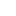喜欢 | 1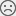不喜欢 | 0

JAVA工程师交流群 +加入

WEB/H5前端交流群 +加入•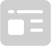资料索取
•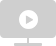答疑解惑
•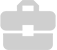技术交流
•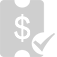职业测评
•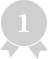面试技巧
•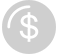高薪秘籍
Python30天热搜词 更多>>
X